# The formation constant of M(CN),1'-is 2.50 x 101, where M is a generic metal A 0.130...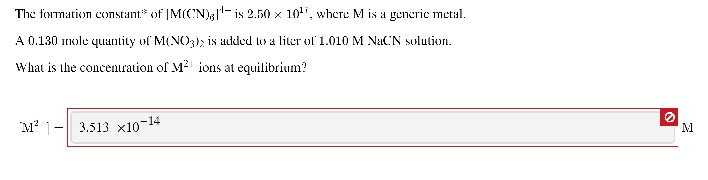The formation constant of M(CN),1'-is 2.50 x 101, where M is a generic metal A 0.130 wole quantity of M(NO3)> is alled to a liter of' 1.010 M NaCN solution. What is the concentration of Mº ions at equilibrium? M? 1- 3.513 x10-14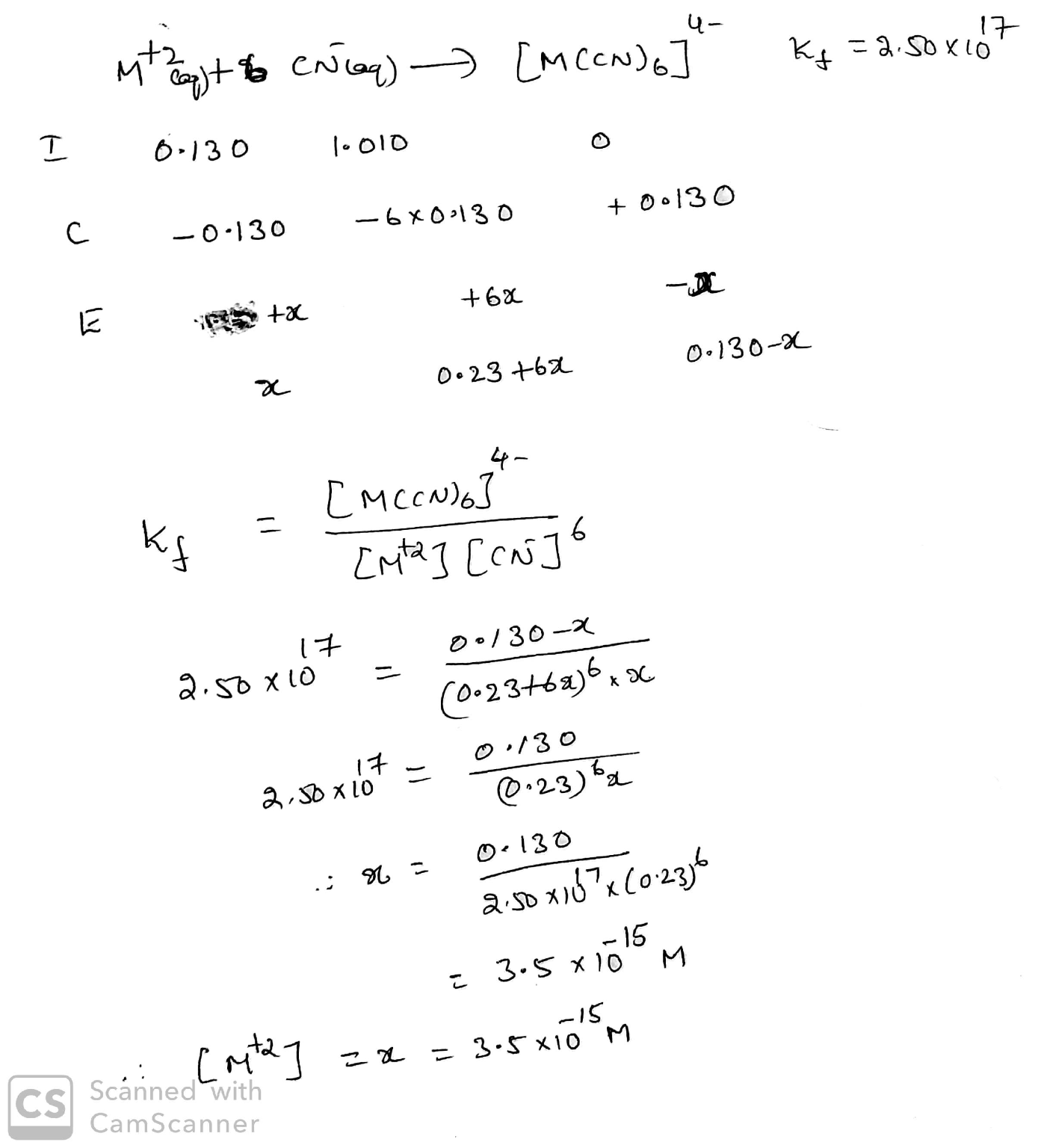#### Earn Coin

Coins can be redeemed for fabulous gifts.

Similar Homework Help Questions
• ### The formation constant of M(CN)614-is 2.50 x 10'7, where M is a generic metal. A 0.130...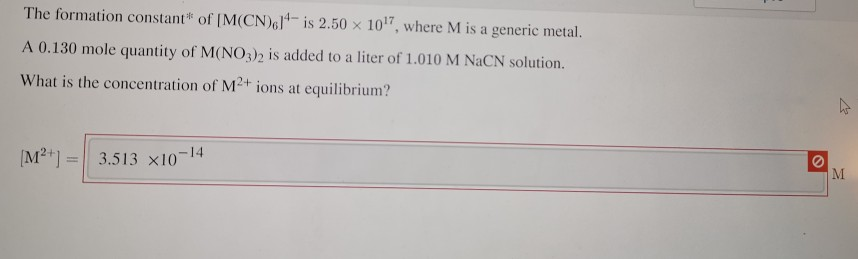The formation constant of M(CN)614-is 2.50 x 10'7, where M is a generic metal. A 0.130 mole quantity of M(NO3)2 is added to a liter of 1.010 M NaCN solution. What is the concentration of M2+ ions at equilibrium? [M²+] = 3.513 x10-14

• ### The formation constant* of [M(CN)6]4– is 2.50 × 1017, where M is a generic metal. A...

The formation constant* of [M(CN)6]4– is 2.50 × 1017, where M is a generic metal. A 0.170-mole quantity of M(NO3)2 is added to a liter of 1.41 M NaCN solution. What is the concentration of M2 ions at equilibrium?

• ### The formation constant* of [M(CN) 4 ]2− is 7.70×1016 , where M is a generic metal....

The formation constant* of [M(CN) 4 ]2− is 7.70×1016 , where M is a generic metal. A 0.130 mole quantity of M(NO3)2 is added to a liter of 0.870 M NaCN solution. What is the concentration of M2+ ions at equilibrium?

• ### The formation constant* of [M(CN)2]– is 5.30 × 1018, where M is a generic metal. A...

The formation constant* of [M(CN)2]– is 5.30 × 1018, where M is a generic metal. A 0.170-mole quantity of M(NO3) is added to a liter of 0.540 M NaCN solution. What is the concentration of M ions at equilibrium?

• ### The formation constant* of [M(CN) 6 ]4− is 2.50 × 10 17 , where M is...

The formation constant* of [M(CN) 6 ]4− is 2.50 × 10 17 , where M is a generic metal. A 0.140 mole quantity of M(NO3)2 is added to a liter of 1.210 M NaCN solution. What is the concentration of M2+ ions at equilibrium?

• ### The formation constant* of [M(CN)2]-is 5.30 x 1018, where M is a generic metal. A 0.170...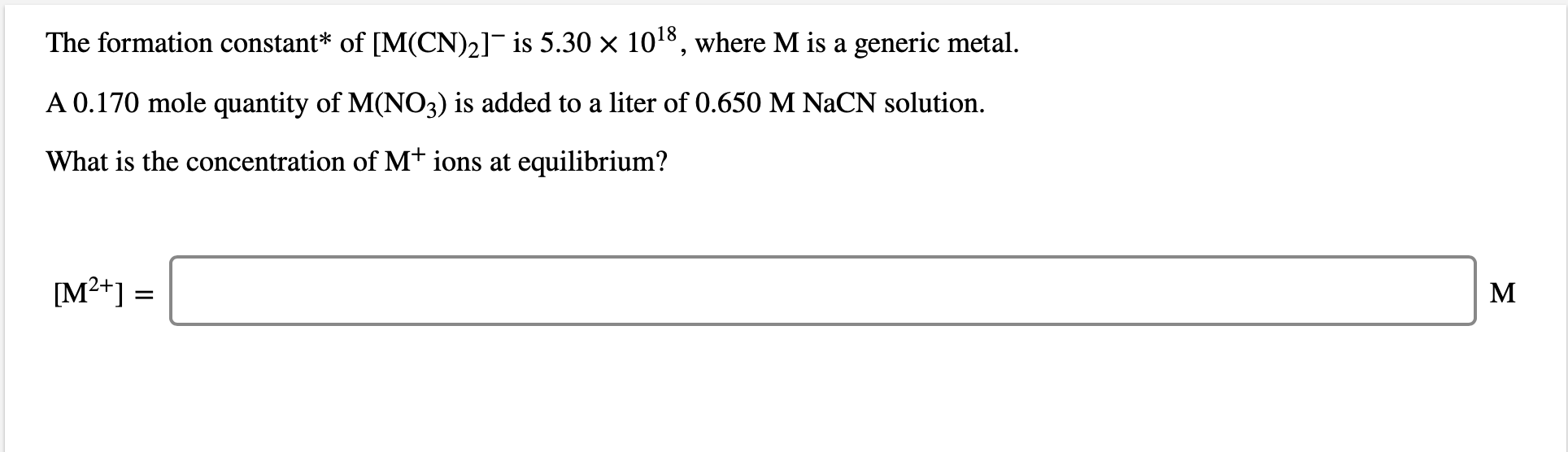The formation constant* of [M(CN)2]-is 5.30 x 1018, where M is a generic metal. A 0.170 mole quantity of M(NO3) is added to a liter of 0.650 M NaCN solution. What is the concentration of M+ ions at equilibrium? [M2+] =

• ### The formation constant* of [M(CN)4]2– is 7.70 × 1016, where M is a generic metal. A...

The formation constant* of [M(CN)4]2– is 7.70 × 1016, where M is a generic metal. A 0.170-mole quantity of M(NO3)2 is added to a liter of 0.930 M NaCN solution. What is the concentration of M2 ions at equilibrium?

• ### can someone please help me answer these, for some reason theh are both wrong and i cant get them right, will rate y...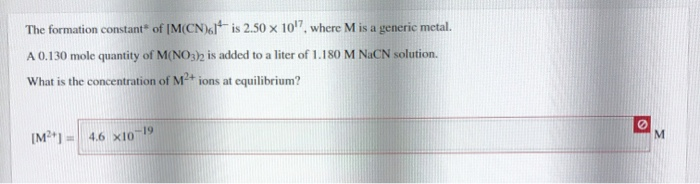can someone please help me answer these, for some reason theh are both wrong and i cant get them right, will rate you good if you can help get both correct answers! thanks The formation constant of M(CN)61 is 2.50 x 10'7, where M is a generic metal. A 0.130 mole quantity of M(NO3) is added to a liter of 1.180 M NaCN solution. What is the concentration of M2+ ions at equilibrium? [M ] = 4.6 x10-19 The generic...

• ### Concentration at equilibrium?

The formation constant* of #[M(CN)_6]^(4– #is #2.50 × 10^17#, where M is a generic metal. A 0.150-mole quantity of #M(NO_3)^2 #is added to a liter of 1.220M NaCN solution. What is the concentration of #M^(2+)# ions at equilibrium?

• ### The formation constant, Kf, of Fe(CN)63-(aq), Fe3+(aq) + 6 CN-(aq) ⇌ Fe(CN)63-(aq), is 1.0 x 1042....

The formation constant, Kf, of Fe(CN)63-(aq), Fe3+(aq) + 6 CN-(aq) ⇌ Fe(CN)63-(aq), is 1.0 x 1042. What are the equilibrium concentrations of Fe3+(aq), CN-(aq), and Fe(CN)63-(aq) if we add 0.113 mol of Fe(NO3)3 to 1.000 L of a 0.965 M aqueous solution of NaCN? Assume the volume remains fixed at 1.000 L.